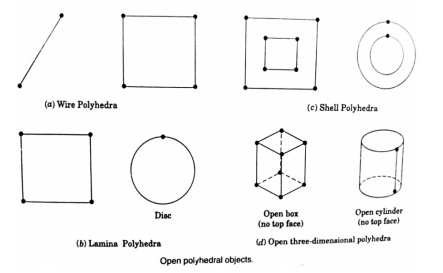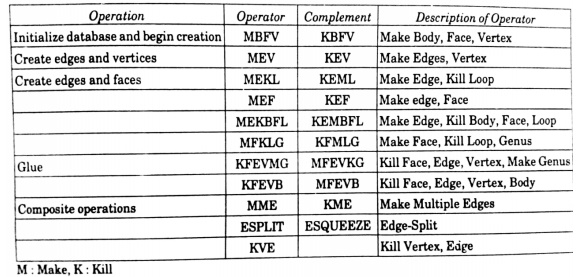Home | | Computer Aided Design and Manufacturing | Boundary representation method (B-rep) and Constructive Solid Geometry (CSG and C-rep)

# Boundary representation method (B-rep) and Constructive Solid Geometry (CSG and C-rep)

Solid Modeling Techniques The various methods for representing the solids are: 1. Half-space m ethod 2. Boundary rep resentation method (B-rep) 3. Constructive solid geometry (CSG and C-rep) 4. Sweep repres entation 5. Analytical solid modeling (ASM) 6. Primitive inst ancing 7. Spatial partiti oning representation a. Cell d ecomposition b. Spatial occupancy enumeration c. Octree encoding

Solid Modeling Techniques

The various methods for representing the solids are:

1.     Half-space m ethod

2.     Boundary rep resentation method (B-rep)

3.            Constructive solid geometry (CSG and C-rep)

4.            Sweep repres entation

5.            Analytical solid modeling (ASM)

6.            Primitive inst ancing

7.            Spatial partiti oning representation

a.     Cell d ecomposition

b.     Spatial occupancy enumeration

c.      Octree encoding

Boundary representation method (B-rep)The main topological ite ms / primitives of b-rep are:

In solid modeling and computer-aided design, boundary representation often abbreviated as B-rep or BREP—is a method for representing shapes using the limits.

A solid is represented as a collection of connected surface elements, the boundary between solid and non-so lid.

Boundary representation models are composed of two parts:

O  Topology, and

O  Geometry (surfaces, curves and points).

Vertex (V) : It is a unique point (an ordered triplet) in space

Edge (E): It is finite, non-self intersecting, directed space c urve bounded by t wo vertices that are not necessarily distinct

Face (F) : It is defined as a finite connected, non-self-intersecting, region of a closed oriented surface bounded by one or mor e loops

Loop (L) : It is an ordered alternating sequence of vertices and edges

Genus (G) : It is the topological name for the number of handles or through holes in an object

Body/Shell(B) : It is a set of faces that bound a single connected c losed volume. A m inimum body is a point

A minimum body is a p oint; topologically this body has one face, one vertex, and no edges. It is called a semin al or singular body.

Geometry

Open polyhedral objectsCurved ObjectsEuler’s formula

Euler – Poinc are Law for closed objects : F – E + V – L = 2 (B – G)

Euler – Poinc are Law for open objects : F – E + V – L =  B  – GSome Euler OperationsSolid Model Generation using B-repAppropriate to construct solid models of unusual shapes

Relatively simple to c onvert a b-rep model to wireframe model

Requires more storage

Not suitable for applications like tool path generation

Slow manipulation

Constructive Solid Geometry (CSG and C-rep)

Constructive solid geometry (CSG) (formerly called computational binary solid geometry) is a techni que used in solid modeling.

Constructive solid ge ometry allows a modeler to create a complex surface or object by using Boolean ope rators to combine objects.

Often CSG presents a model or surface that appears visually comple x, but is actually little more than cleve rly combined or de-combined objects

The  simplest  solid  objects  used  for  the  representation  are  c alled primitives.

Typically they are the objects of simple shape:

O  cuboids

O  cylinders

O  prisms

O  pyramids

O  spheres

O  conesThe set of allowable primitives is limited by each software packag e. Some software packages allow CSG on curved objects while other packages do not

It is said that an object is constructed from primitives by means of allowable operations , which are typically Boolean o perations on sets: union, intersection an d difference, as well as geometric transformati ons of those sets

Boolean OperationsCSG TreeStudy Material, Lecturing Notes, Assignment, Reference, Wiki description explanation, brief detail
Mechanical : Computer Aided Design : Geometric Modeling : Boundary representation method (B-rep) and Constructive Solid Geometry (CSG and C-rep) |